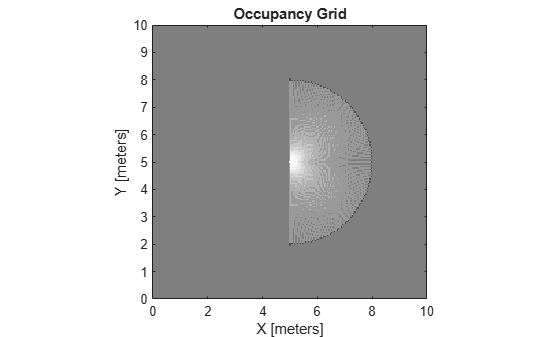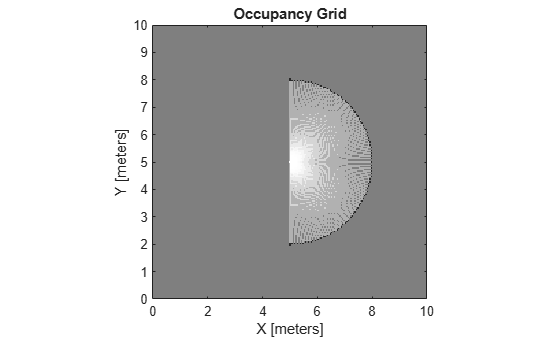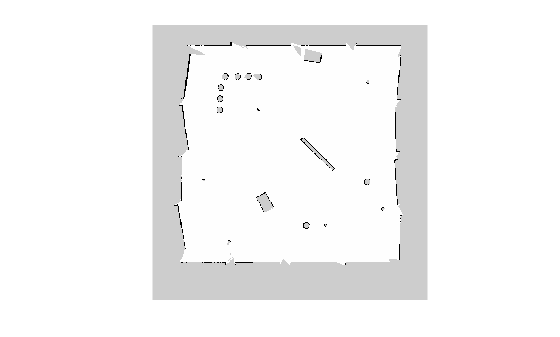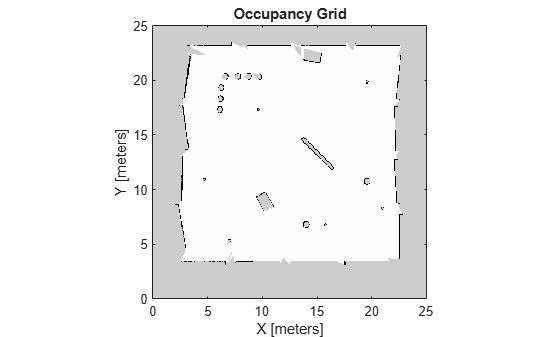# occupancyMap

Create occupancy map with probabilistic values

## Description

`occupancyMap` creates a 2-D occupancy grid map object. Each cell in the occupancy grid has a value representing the probability of the occupancy of that cell. Values close to 1 represent a high probability that the cell contains an obstacle. Values close to 0 represent a high probability that the cell is not occupied and obstacle free.

Occupancy maps are used in navigation algorithms such as path planning (see `plannerRRT`). They are also used in mapping applications for finding collision-free paths, performing collision avoidance, and calculating localization (see `monteCarloLocalization`). You can modify your occupancy map to fit your specific application.

The `occupancyMap` objects support local coordinates, world coordinates, and grid indices. The first grid location with index `(1,1)` begins in the top-left corner of the grid.

Use the `occupancyMap` class to create 2-D maps of an environment with probability values representing different obstacles in your world. You can specify exact probability values of cells or include observations from sensors such as laser scanners.

Probability values are stored using a binary Bayes filter to estimate the occupancy of each grid cell. A log-odds representation is used, with values stored as `int16` to reduce the map storage size and allow for real-time applications.

## Creation

### Syntax

``map = occupancyMap(width,height)``
``map = occupancyMap(width,height,resolution)``
``map = occupancyMap(rows,cols,resolution,'grid')``
``map = occupancyMap(p)``
``map = occupancyMap(p,resolution)``
``map = occupancyMap(sourcemap)``
``map = occupancyMap(sourcemap,resolution)``

### Description

````map = occupancyMap(width,height)` creates a 2-D occupancy map object representing a world space of `width` and `height` in meters. The default grid resolution is 1 cell per meter.```

example

````map = occupancyMap(width,height,resolution)` creates an occupancy map with a specified grid resolution in cells per meter. `resolution` sets the Resolution property.```
````map = occupancyMap(rows,cols,resolution,'grid')` creates an occupancy map with the specified number of rows and columns and with the resolution in cells per meter. The values of `rows` and `cols` sets the GridSize property.```
````map = occupancyMap(p)` creates an occupancy map from the values in matrix `p`. The grid size matches the size of the matrix, with each cell probability value interpreted from the matrix location.```

example

````map = occupancyMap(p,resolution)` creates an occupancy map from the specified matrix and resolution in cells per meter.```
````map = occupancyMap(sourcemap)` creates an object using values from another `occupancyMap` object.```
````map = occupancyMap(sourcemap,resolution)` creates an object using values from another `occupancyMap` object, but resamples the matrix to have the specified resolution.```

### Input Arguments

expand all

Map width, specified as a scalar in meters.

Map height, specified as a scalar in meters.

Grid resolution, specified as a scalar in cells per meter.

Number of rows in grid, specified as a positive scalar integer.

Number of columns in grid, specified as a positive scalar integer.

Input occupancy grid, specified as a matrix of probability values from `0` to `1`. The size of the grid matches the size of the matrix. Each matrix element corresponds to the probability of the grid cell location being occupied. Values close to `1` represent a high certainty that the cell contains an obstacle. Values close to `0` represent certainty that the cell is not occupied and obstacle free.

Occupancy map object, specified as a `occupancyMap` object.

## Properties

expand all

Threshold below which cells are considered obstacle-free, specified as a scalar between `0` and `1` inclusive. Cells with probability values below this threshold are considered obstacle free. This property also defines the free locations for path planning when using objects like `plannerRRT`.

Data Types: `double`

Threshold above which cells are considered occupied, specified as a scalar. Cells with probability values above this threshold are considered occupied.

Data Types: `double`

Saturation limits for probability, specified as a 1-by-2 real-valued vector representing the minimum and maximum values, in that order. Values above or below these saturation values are set to the minimum and maximum values. This property reduces oversaturating of cells when incorporating multiple observations.

Data Types: `double`

Number of rows and columns in grid, stored as a 1-by-2 real-valued vector representing the number of rows and columns, in that order.

Data Types: `double`

Grid resolution, stored as a scalar in cells per meter representing the number and size of grid locations.

Data Types: `double`

Minimum and maximum values of x-coordinates in local frame, stored as a two-element horizontal vector of the form ```[min max]```. Local frame is defined by `LocalOriginInWorld` property.

Data Types: `double`

Minimum and maximum values of y-coordinates in local frame, stored as a two-element horizontal vector of the form ```[min max]```. Local frame is defined by `LocalOriginInWorld` property.

Data Types: `double`

Minimum and maximum world range values of x-coordinates, stored as a 1-by-2 vector representing the minimum and maximum values, in that order.

Data Types: `double`

Minimum and maximum world range values of y-coordinates, stored as a 1-by-2 vector representing the minimum and maximum values, in that order.

Data Types: `double`

[x,y] world coordinates of the bottom-left corner of the grid, specified as a 1-by-2 vector.

Data Types: `double`

Location of the origin of the local frame in world coordinates, specified as a two-element vector, `[xLocal yLocal]`. Use the `move` function to shift the local frame as your vehicle moves.

Data Types: `double`

Location of the bottom-left corner of the grid in local coordinates, specified as a two-element vector, ```[xLocal yLocal]```.

Data Types: `double`

Default value for unspecified map locations including areas outside the map, specified as a scalar between `0` and `1` inclusive.

Data Types: `double`

## Object Functions

 `checkOccupancy` Check locations for free, occupied, or unknown values `copy` Create copy of occupancy grid `getOccupancy` Get occupancy value of locations `grid2local` Convert grid indices to local coordinates `grid2world` Convert grid indices to world coordinates `inflate` Inflate each occupied grid location `insertRay` Insert ray from laser scan observation `local2grid` Convert local coordinates to grid indices `local2world` Convert local coordinates to world coordinates `move` Move map in world frame `occupancyMatrix` Convert occupancy grid to double matrix `raycast` Compute cell indices along a ray `rayIntersection` Find intersection points of rays and occupied map cells `setOccupancy` Set occupancy value of locations `show` Show grid values in a figure `syncWith` Sync map with overlapping map `updateOccupancy` Integrate probability observations at locations `world2grid` Convert world coordinates to grid indices `world2local` Convert world coordinates to local coordinates

## Examples

collapse all

Create an empty occupancy grid map.

`map = occupancyMap(10,10,20);`

Input pose of the vehicle, ranges, angles, and the maximum range of the laser scan.

```pose = [5,5,0]; ranges = 3*ones(100,1); angles = linspace(-pi/2,pi/2,100); maxrange = 20;```

Create a `lidarScan` object with the specified ranges and angles.

`scan = lidarScan(ranges,angles);`

Insert the laser scan data into the occupancy map.

`insertRay(map,pose,scan,maxrange);`

Show the map to see the results of inserting the laser scan.

`show(map)`Check the occupancy of the spot directly in front of the vehicle.

`getOccupancy(map,[8 5])`
```ans = 0.7000 ```

Add a second reading and view the update to the occupancy values. The additional reading increases the confidence in the readings. The free and occupied values become more distinct.

```insertRay(map,pose,scan,maxrange); show(map)````getOccupancy(map,[8 5])`
```ans = 0.8448 ```

Convert a portable graymap (PGM) file containing a ROS map into an `occupancyMap` for use in MATLAB.

Import the image using `imread`. Crop the image to the playpen area.

```image = imread('playpen_map.pgm'); imageCropped = image(750:1250,750:1250); imshow(imageCropped)```PGM values are expressed from 0 to 255 as `uint8`. Normalize these values by converting the cropped image to `double` and dividing each cell by 255. This image shows obstacles as values close to 0. Subtract the normalized image from 1 to get occupancy values with 1 representing occupied space.

```imageNorm = double(imageCropped)/255; imageOccupancy = 1 - imageNorm;```

Create the `occupancyMap` object using an adjusted map image. The imported map resolution is 20 cells per meter.

```map = occupancyMap(imageOccupancy,20); show(map)```## Limitations

Occupancy values have a limited resolution of ±0.001. The values are stored as `int16` using a log-odds representation. This data type limits resolution, but saves memory when storing large maps in MATLAB®. When calling `setOccupancy` and then `getOccupancy`, the value returned might not equal the value you set. For more information, see the log-odds representations section in Occupancy Grids.

If memory size is a limitation, consider using `binaryOccupancyMap` instead. The binary occupancy map uses less memory with binary values, but still works with Navigation Toolbox™ algorithms and other applications.

## Extended Capabilities

### C/C++ Code GenerationGenerate C and C++ code using MATLAB® Coder™.

Introduced in R2019b